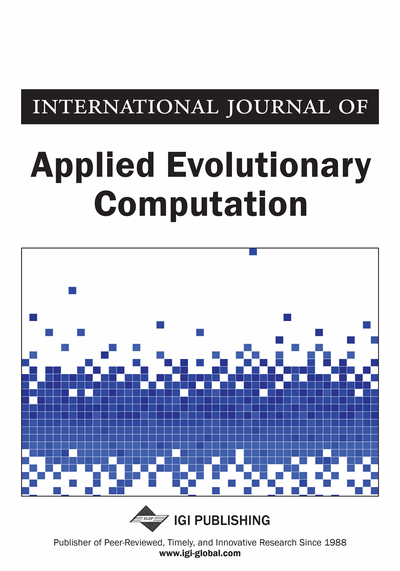# Ant Colony Algorithms for Data Learning

Mohamed Hamlich (Computer Science Lab, UH2, FSTM, Mohammadia, Morocco) and Mohammed Ramdani (Computer Science Lab, UH2, FSTM, Mohammadia, Morocco)
DOI: 10.4018/jaec.2013070101

## Abstract

Fuzzy Ant-Miner algorithm processes data with nominal class and has the disadvantage of not treating the data with continuous class. In this paper, after presenting the Fuzzy Ant Miner algorithm, the authors propose a new learning method to partition heterogeneous data with continuous class. This method in a first step finds the optimal path between the data using algorithms of ants. Distance adopted in their optimization method takes into account all types of data. The second step vise to divide the data into homogeneous groups by browsing the optimal path found. A new test probability is estimated based on the distance and the amount of pheromone deposited by ants in the transitions between the data. A third step is to find the prototype of each cluster to identify the cluster membership of any new data injected.
Article Preview
Top

## 2. Fuzzy Ant-Miner Algorithm

### 2.1. Fuzzy Discretization

In order to avoid the crisp discretization used by cAnt-Miner (Otero, Freitas, & Johnson, 2008), our method extends the crisp partition by discretizing the values of an attribute into fuzzy partitions. First a threshold x is determined in the same way as cAnt-Miner (the one that leads to the minimum entropy (Quinlan, 1993)). We assume that the threshold is a fuzzy number around x. Then the values of continuous attribute are transformed into membership degrees of the fuzzy values Ai(low)<< x, Ai(average)≈ x and Ai(high)>> x. Where the membership functions of the continuous attributeto a fuzzy value Ai<< x is calculated by Figure 1:=(1)

The membership functions of the continuous attribute Ai to a fuzzy value Aix is calculated by:=(2)

The membership functions of the continuous attribute Ai to a fuzzy value Ai>> x is calculated by:=(3)

Where parameters (a, b, c et d) determine the boundaries of the fuzzy area and so the degree of fuzziness. The user sets these parameters for the best accuracy. Note that other ways of generating the fuzzy partitions Liu, Hussain, Tan, & Dash, 2002, Marsala, 1998 can be used in our method.

## Complete Article List

Search this Journal:
Reset
Open Access Articles: Forthcoming
Volume 12: 4 Issues (2021): Forthcoming, Available for Pre-Order
Volume 11: 4 Issues (2020): 3 Released, 1 Forthcoming
Volume 10: 4 Issues (2019)
Volume 9: 4 Issues (2018)
Volume 8: 4 Issues (2017)
Volume 7: 4 Issues (2016)
Volume 6: 4 Issues (2015)
Volume 5: 4 Issues (2014)
Volume 4: 4 Issues (2013)
Volume 3: 4 Issues (2012)
Volume 2: 4 Issues (2011)
Volume 1: 4 Issues (2010)
View Complete Journal Contents Listing